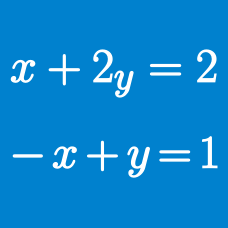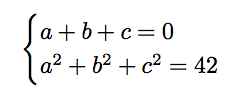Algebra

# System of Equations - Problem Solving

$$OAB$$ is a triangle in the first quadrant of the xy-plane with vertices $$O(0,0)$$, $$A(5,0)$$ and $$B(x,y)$$, where $$x$$ and $$y$$ are positive real numbers. If $$OB = 6$$ and $$AB = 3$$, then $$y^2$$ can be written as $$\frac{a}{b}$$, where $$a$$ and $$b$$ are coprime positive integers, what is the value of $$a+b$$?What is $$a^4 + b^4 + c^4$$?

In a school, there are $$4$$ more students in Class A than in Class B, and $$4$$ more students in Class B than in Class C. As a reward for good behavior, a teacher decides to give out some sweets. Every student in class C got $$5$$ more sweets than every student in Class B, and every student in Class B got $$3$$ more sweets than every student in Class A. As it turns out, Class A got $$10$$ more sweets than Class B, and Class B got $$14$$ more sweets than Class C. How many sweets were given out in all?

Given the system of equations $\begin{cases} x(x+y) &=& 9 \\ y(x+y) &=& 16 \end{cases}$ the value of $$xy$$ can be written as $$\frac{a}{b}$$ where $$a$$ and $$b$$ are positive coprime integers. Find $$a+b$$.

An ordered triple of real numbers $$(a, b, c)$$ is called friendly, if each number is equal to the product of the other 2. How many (distinct) friendly triples are there?

Details and assumptions

The numbers need not be pairwise distinct (which means that no two of them are the same).

×